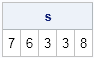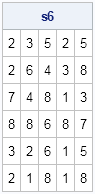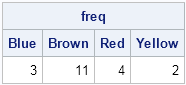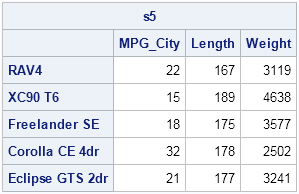With each release of SAS/IML software, the language provides simple ways to carry out tasks that previously required more effort. In 2010 I blogged about a SAS/IML module that appeared in my book Statistical Programming with SAS/IML Software, which was written by using the SAS/IML 9.2. The blog post showed how to sample with replacement (with equal probability) from a finite set of possibilities.

As of SAS/IML 12.1, there is a built-in function that returns random samples from a finite set. The SAMPLE function makes it easy to do the following:

• Sample with replacement with equal or varying probabilities
• Sample without replacement
• Generate multiple samples with a single call

Sampling with replacement is a common task for bootstrap (resampling) methods, so let's start by discussing sampling with replacement.

### Sample with replacement with equal probability

You can use the SAMPLE function in the SAS/IML language to sample with replacement from a finite set. In my 2010 article, I used the example of choosing five elements at random from the set {1, 2, ..., 8}. The following call shows how to use the built-in SAMPLE function for this task:

```proc iml; call randseed(1234); s = sample(1:8, 5); /* randomly choose 5 elements from the set 1:8 */ print s;```The default sampling scheme is to sample with replacement, which is why the element 3 appears twice in the random sample. Notice that the random number seed for the SAMPLE function is set by using the RANDSEED subroutine.

If you want to generate multiple samples, each of size five, you can specify a two-element vector for the second argument. The first element specifies the sample size. The second element specifies the number of samples, which is the number of rows in the output matrix. For example, the following statement generates six random samples. Each row is one random sample and contains five elements.

```s6 = sample(1:8, {5 6}); /* sample size=5; number of samples=6 */ print s6;```### Sample with replacement with varying probabilities

The SAMPLE function also supports sampling with unequal probabilities. Since SAS is known for having free M&Ms® in the breakrooms, here's an M&M-inspired example. There is a large jar of plain M&Ms in my breakroom. The M&Ms are different colors: 30% are brown, 20% are yellow, 20% are red, 10% are green, 10% are orange, and 10% are blue. I'll use the SAMPLE function to simulate drawing 20 M&Ms from the jar. Although in real life I would never select an M&M and then replace it back into the jar (Yuck! Unsanitary!), the jar is so large that the probabilities are approximately constant during the sampling, so I can use the sampling with replacement method.

```colors = {"Brown" "Yellow" "Red" "Green" "Orange" "Blue"}; prob = {0.3 0.2 0.2 0.1 0.1 0.1}; snack = sample(colors, 20, "Replace", prob); /* a 1x20 vector of colors */ call tabulate(category, freq, snack); /* count how many of each color */ print freq[colname=category];```For this sample of 20 candies, more than half of the sample is brown; I did not draw any greens or oranges. The output of the SAMPLE function is a 1 x 20 vector of colors. If I only want the total number of each color—and not the sample itself—I could use the RANDMULTINOMIAL function to simulate the counts directly, rather than use the SAMPLE function and the TABULATE subroutine.

### Sampling observations in a data matrix

If you have data in a SAS/IML matrix, you can sample the observations by sampling from the integers 1, 2, ..., N, where N is the number of rows of the matrix. For example, the following statements read in 428 observations from the Sashelp.Cars data set. The SAMPLE function is used to draw a random sample that contains five observations. Each observation contains information about a random vehicle in the data set.

```proc iml; call randseed(1); use Sashelp.Cars; varNames = {"MPG_City" "Length" "Weight"}; read all var varNames into x[rowname=Model]; close Sashelp.Cars;   obsIdx = sample(1:nrow(x), 5); /* sample size=5, rows chosen from 1:NumRows */ s5 = x[obsidx, ]; /* extract subset of rows */ print s5[rowname=(Model[obsIdx]) colname=varNames];```In summary, this article has shown how to use the SAMPLE function in SAS/IML 12.1 to sample with replacement from a finite set. In future posts I will show how to use other SAS tools to resample from a data set and how to sample without replacement.

Share